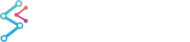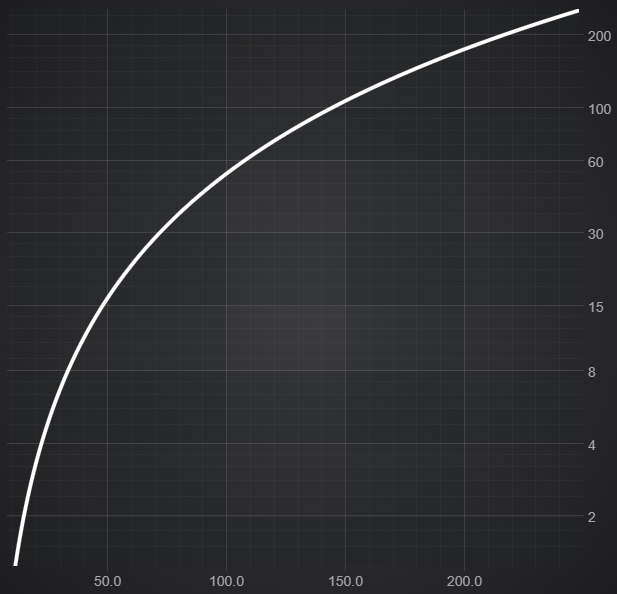﻿ Logarithmic Axis | JavaScript Chart DocumentationSciChart.js JavaScript 2D Charts API > Axis APIs > Logarithmic Axis
Logarithmic Axis

The LogarithicAxis is a Value axis similar to the normal NumericAxis, but where the tick values increase exponentially. Plotting data on such an axis is equivalent to plotting the log of that data. You can set the logarithmic base using the logBase property. eg logBase: 10 (the default) will result in ticks like 1, 10, 100, 1000. logBase 2 will result in ticks like 2, 4, 8, 16, 32.

NumericAxis
Copy Code
import {SciChartSurface} from "scichart/Charting/Visuals/SciChartSurface";
import {EAxisAlignment} from "scichart/types/AxisAlignment";
import {ENumericFormat} from "scichart/types/NumericFormat";
import {LogarithmicAxis} from "scichart/Charting/Visuals/Axis/LogarithmicAxis";
import {NumberRange} from "scichart/Core/NumberRange";
axisTitle: "Log(10) Axis",
axisAlignment: EAxisAlignment.Bottom,
labelFormat: ENumericFormat.Scientific,
logBase: 10,
visibleRange: new NumberRange(1, 1E6)
}));

When using logarithmicAxis and the labelFormat ENumericFormat.Scientific, the logBase of the axis will be used as the base for the label. This is NOT the case for ENumericFormat.Exponential which is always base 10.

LogarithmicAxis cannot show both positive and negative numbers on the same axis, so if your data is negative you need to set isNegative on the axis.  If you need to show positive and negative log data, you need to split it into positive and negative sets and plot them on seperate vertically stacked axes.

Minor Tick Mode

By default, Major gridlines are spaced logarithmically, and Minor gridlines are spaced linearly between them. If your visible range is extremely large, you may want to switch to logarithmic spacing for minor gridlines, which you can do with the minorTickMode property which is an ELogarithmicMinorTickMode which can be Logarithmic, Linear or Auto

Auto mode means it switches from linear to Logarithmic when the visible range is such that the first linear minor tick would be more than 70% of the major tick

Major Tick Mode - Financial Log Charts

For financial charts you often want base 2, with a relatively small range, but you don't want your tick labels to be powers of 2. In this case set majorTickMode to ELogarithmicMajorTickMode.RoundNumbers. This will give you labels with nice round numbers, at the expense of gridlines that are not exactly equally spaced.

labelFormat: ENumericFormat.SignificantFigures is also helpful as it retains precision for very small values, while not resulting in unnecessary decimal places for large values.

LogarithmicAxis
Copy Code
import {SciChartSurface} from "scichart/Charting/Visuals/SciChartSurface";
import {EAxisAlignment} from "scichart/types/AxisAlignment";
import {ENumericFormat} from "scichart/types/NumericFormat";
import {LogarithmicAxis} from "scichart/Charting/Visuals/Axis/LogarithmicAxis";
import {NumberRange} from "scichart/Core/NumberRange";
import { ELogarithmicMajorTickMode, ELogarithmicMinorTickMode } from "scichart/Charting/Numerics/TickProviders/LogarithmicTickProvider";
// Creating a Log(2) Axis on the YAxis